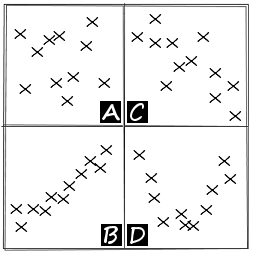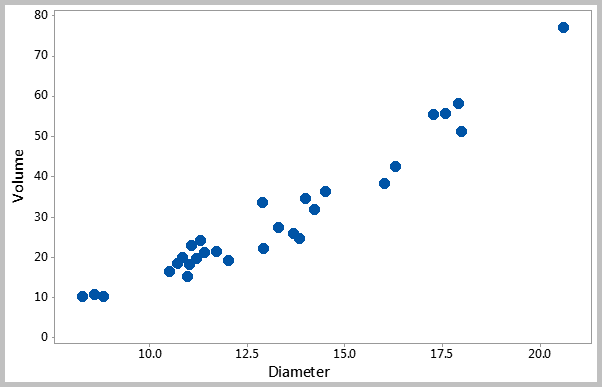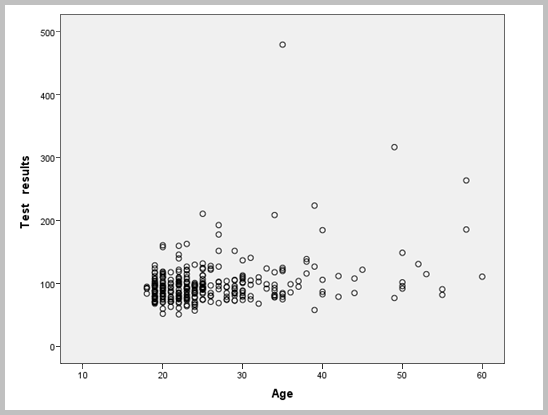# Scatter PlotsMany situations require the investigating whether a relationship exists between two or more variables. A Scatter Plot is a diagram showing whether two variables are correlated or related to each other. It shows patterns in the relationship that cannot be seen by just looking at the data. It is often used as a first step in analyzing and communicating the correlation between pairs of variables before conducting advanced statistical analyses. It works with both continuous and count data.

A line manager for example may want to check the relationship between the number of training hours and employee productivity, or if the number of defects is a function of the experience of the person doing it. He may then be interested in studying the relationship between equipment downtime and its cost of maintenance. Other examples:

• The relationship between driving speed and fuel consumption.
• The relationship between the number of people working on a shift and the average answer time in a call center.
• The relationship between the number of years of education someone has and the annual income of that person.

A scatter plot is primarily used to visually investigate the relationship between two variables (often an output and an input variables). This is useful to verify that a change in one variable can affect the other variable. It helps detecting the primary factors that are really causing a problem and hence eliminating non-critical factors from consideration. It can also determine the strength of the relationship between the variables. It is often used with statistical tools (such as regression) to support or reject hypotheses about the data.

## How to Construct a Scatter Plot:

• You must first collect the two paired sets of data.
• Once you have collected enough data points, create a summary table of the data.
• Draw and label the horizontal and vertical axes with variable names and scale values.
• Plot the data pairs on the diagram by placing a dot at the intersection of each data pair.
• Look at how the pattern appears and how the two variables vary together. Note that the width of the scattered pattern reflects the strength of the relationship.

Scatter plots are more useful when comparing an input variable with an output variable which will help identifying the factors that are causing problems to a process. The independent variable (or the explanatory variable) is normally placed on the horizontal axis while the dependent variable (or the response variable) is placed on the vertical axis. You may also compare two input or output variables to each other. In this case, it doesn’t matter which variable goes on the horizontal axis and which goes on the vertical axis.Scatter plots can indicate several types of correlation:

• There may be no correlation at all when the data points are scattered randomly without showing any particular pattern.
• A positive correlation occurs when the values of one variable increase as the values of the other also increase (or when the fitted line slopes from bottom left to top right).
• A negative correlation occurs when the values of one variable increase as the values of the other decrease (or when the fitted line slopes from upper left down to lower right).
• Scatter plots can also indicate nonlinear relationships between variables.
The following scatter plot shows the relationship between the volume and the diameter of sample trees in a forest. Note that both variables are output variables.The above chart shows the results of a data set that belongs to Minitab Inc.

## Matrix Plots:

A Matrix Plot is used to summarize the relationship between several variables by producing a scatter plot for every combination of variables in the data set. It allows to visually assess the variables that might be related in some way. Potential correlations can then be identified for further investigation.

The following is a matrix plot that shows the relationships between pairs of variables all at once in one graph. It appears that there is some relationship between the years of experience and the salaries. However, the number of publications does not appear to be correlated with the years of experience.The above chart shows the results of a data set that belongs to Minitab Inc.

## Further Information:

• When the relationship is not so clear, Correlation can be used to help determine if a relationship exists between the variables. Regression techniques go a step further by defining the relationship in a mathematical format.
• Be careful before concluding that there is a direct cause-and-effect relationship between the variables. There might be a third factor that is causing the change in the two variables.
• You can also illustrate a stratification factor in the scatter plot. For example, the relationship between a process output and a process input for two different settings.

Related Resources